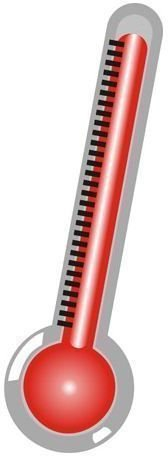Lesson Plan Measuring Energy Changes in Chemical Reactions

Prelab Discussion

Before beginning the lab, have the students read through it in its entirety. Then discuss the following:

1. Discuss how a thermometer can be used to measure energy changes.
2. Ask students why the temperature is measured five separate times for each mixture.
3. Ask students to suggest the reason for using foam cups instead of glass beakers or other types of containers.

Always be sure to lay out safety rules when working on science experiments.

Materials and Preparation

Collect the following materials for each student or pair of students:• copy of data table
• labels
• calcium chloride
• baking soda
• 3 plastic or foam cups
• 2 spoons
• water
• thermometer
• timer or clock
• paper towels
• graph paper
• pencil
• three different colored pencils

Label one cup baking soda, one cup calcium chloride, and one cup baking soda + calcium chloride + water.

Procedure

1. Measure 15 mL of water in the graduated cylinder and add it to the baking soda cup.
2. Place the thermometer in the cup.
3. Measure one spoonful of baking soda and place it in the cup with the water.
4. Immediately measure the temperature of the combined reactants and record it in the data table.
5. Continue to measure the temperature at one minute intervals for five minutes. Record your temperature measurements in the data table.
6. After five minutes, remove the thermometer and wipe it clean with a paper towel.
7. Repeat steps 1-6 for calcium chloride + water and for baking soda + calcium chloride + water.
8. On the graph paper, prepare a line graph with temperature on the vertical axis and time on the horizontal axis. Graph the temperature measurements for all three chemical reactions on the same graph, using a different colored pencil for each one. Be sure to make a key so that you know what each color represents.

Analysis

1. Which chemical reaction gave off the most energy? (calcium chloride and water) How could you tell? (The combination produced the highest temperature.)
2. Which chemical reaction absorbed the most energy? (baking soda and water) How could you tell? (the mixture cools)
3. Describe the graph for the chemical reaction that contained both baking soda and calcium chloride. What does this tell you about the chemical reaction? (Answers will vary, but should reflect the information on the graph.)
4. Identify the independent and dependent variables in this activity. (independent variables: substances mixed together; dependent variable: temperature change)

Conclusion

• Write a short paragraph explaining how energy changes relate to chemical reactions.

For an extension project, have students test each of the three chemical reactions to find out how much gas each produces. Conduct each of the chemical reactions in self-lock plastic bags. Try to write simple chemical equations for each of the reactions. Use the equations to reveal the gas produced.

For some guidance in helping your students learn how to apply the scientific method, read "Teaching the Scientific Method".